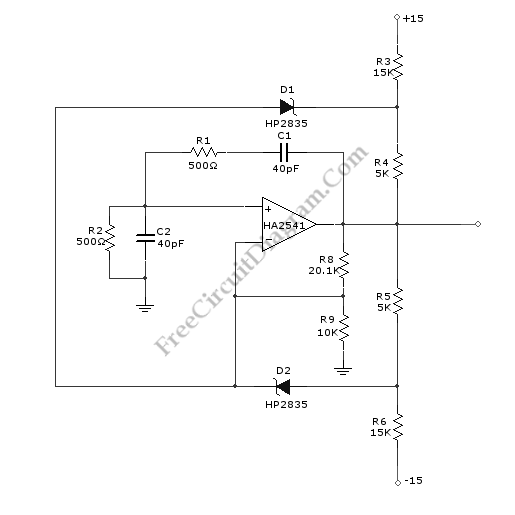# Wien-Bridge Oscillator Using HA2541 Op-AmpA Wien-Bridge oscillator circuit can be built from HA2541 and some basic components. This circuit can generate good-quality sine wave of 40 MHz with an upper limit of 50 MHz. The R3 through R7 and D2 and D1 provide the diode limiting for this circuit. Here is the schematic diagram of this circuit:For frequency stability, this circuit need a regenerative feedback. The feedback network is formed by R2/C2 and R1/C1. To obtain the oscillation, the gain required is three but practically we need gain over three.  So R9 and R8 is added to this circuit to provide gain over three times.